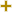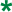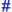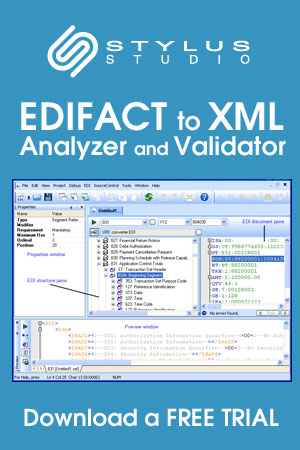Return to Stylus Studio EDIFACT D02A Code List page.

# Calculation sequence codeUN/CEFACT Revision 2002A Code ListRepr:an..3
Desc:Code specifying a calculation sequence.
This table also occurs in the following versions of this standard:
D00A, D00B, D01A, D01B, D01C, D02A, D02B, D03A, D03B, D04A, D04B, D93A, D94A, D94B, D95A, D95B, D96A, D96B, D97A, D97B, D98A, D98B, D99A, D99B

 1 First step of calculation Code specifying the first step of a calculation. 2 Second step of calculation Code specifying the second step of a calculation. 3 Third step of calculation Code specifying the third step of a calculation. 4 Fourth step of calculation Code specifying the fourth step of a calculation. 5 Fifth step of calculation Code specifying the fifth step of a calculation. 6 Sixth step of calculation Code specifying the sixth step of a calculation. 7 Seventh step of calculation Code specifying the seventh step of a calculation. 8 Eighth step of calculation Code specifying the eighth step of a calculation. 9 Ninth step of calculation Code specifying the ninth step of a calculation.

Change indicators
plus signAn addition.
asteriskAddition/substraction/change to a code entry for a particular data element.
hash or pound signChanges to names.
vertical barChanges to text for descriptions, notes and functions.
minus signA deletion.
letter XMarked for deletion.

Usage indicatorsUsed in batch messages only.Common usage in both batch and interactive messages.Used in interactive messages only.

Return to Stylus Studio EDIFACT D02A Code List page.